• 一维数组线性插值
千次阅读
2020-12-09 20:37:29

直接列出函数：

numpy.interp(x, xp, fp, left=None, right=None, period=None)

x - 表示将要计算的插值点x坐标

xp - 表示已有的xp数组

fp - 表示对应于已有的xp数组的值

left - 表示当x值在xp中最小值左边时，x对应y的值为left

right - 表示当x值在xp中最大值右边时，x对应y的值为right

(left和right表示x在xp的域外时，y的取值)

example：

1.

import numpy as np

xp = [1, 2, 3]

fp = [3, 2, 0]

print(np.interp(2.5, xp, fp))

output：1.0

2.

import numpy as np

xp = [1, 2, 3]

fp = [3, 2, 0]

x = [0, 1, 1.5, 2.72, 3.14]

print(np.interp(x, xp, fp))

output：[3. 3. 2.5 0.56 0. ]

3.

import numpy as np

xp = [1, 2, 3]

fp = [3, 2, 0]

x = [0, 1, 1.5, 2.72, 3.14]

print(np.interp(x, xp, fp, -99, 99))

output：[-99. 3. 2.5 0.56 99. ]

补充知识：numpy 的一维插值函数interp

numpy.interp(x, xp, fp, left=None, right=None, period=None)

返回离散数据的一维分段线性插值结果，浮点数或复数(对应于fp值)或ndarray. 插入数据的纵坐标，和x形状相同。

x: 数组，待插入数据的横坐标.

xp: 一维浮点数序列，原始数据点的横坐标，如果period参数没有指定那么就必须是递增的。否则，在使用xp = xp % period正则化之后，xp在内部进行排序.

fp: 一维浮点数或复数序列原始数据点的纵坐标，和xp序列等长.

left: 可选参数，类型为浮点数或复数(对应于fp值)，当x < xp时的插值返回值，默认为fp.

right: 可选参数，类型为浮点数或复数(对应于fp值)，当x > xp[-1]时的插值返回值，默认为fp[-1].

period: None或者浮点数，可选参数. 横坐标的周期. 此参数使得可以正确插入angular x-coordinates. 如果该参数被设定，那么忽略left参数和right参数。

插入横坐标在原函数横坐标范围内

x = 2.5 # 要插入值的横坐标

xp = [1, 2, 3] # 要插入序列的横坐标

fp = [3, 2, 0] # 要插入序列的纵坐标

y = np.interp(x, xp, fp) # 返回插入值的纵坐标 1.0

plt.plot(xp, fp, '-o')

plt.plot(x, y, 'x')

plt.show()

插入横坐标在原函数横坐标外(默认)

x = [0, 1, 1.5, 2.72, 3.14] # 左侧外部默认为原函数最左侧函数值，右侧默认为右侧

xp = [1, 2, 3]

fp = [3, 2, 0]

y = np.interp(x, xp, fp) # array([ 3. ,3. ,2.5 ,0.56, 0. ])

plt.plot(xp, fp, '-o')

plt.plot(x, y, 'x')

plt.show()

插入横坐标在原函数横坐标外(指定)

x = 3.14

xp = [1, 2, 3]

fp = [3, 2, 0]

UNDEF = -99.0

y = np.interp(x, xp, fp, right=UNDEF) # -99.0

plt.plot(xp, fp, '-o')

plt.plot(x, y, 'x')

plt.show()

正弦插值

x = np.linspace(0, 2 * np.pi, 10) # 在0到2pi的范围内均匀取10个点

y = np.sin(x) # sin函数x横坐标对应的y值

xvals = np.linspace(0, 2 * np.pi, 50) # 均匀取50个

yinterp = np.interp(xvals, x, y) # 在映射关系为y的x中插入xvals

plt.plot(x, y, 'o')

plt.plot(xvals, yinterp, '-x')

plt.show()

以上这篇Numpy一维线性插值函数的用法就是小编分享给大家的全部内容了，希望能给大家一个参考，也希望大家多多支持我们。

本文标题: Numpy一维线性插值函数的用法

本文地址: http://www.cppcns.com/jiaoben/python/309194.html

更多相关内容
• 官方文档链接：https://docs.scipy.org/doc/scipy-1.3.0/reference/generated/scipy.interpolate.interp1d.html#scipy.interpolate.interp1dscipy库中可以通过interp1d类来实现一维插值照例还是官方文档的翻译与解释...

官方文档链接：https://docs.scipy.org/doc/scipy-1.3.0/reference/generated/scipy.interpolate.interp1d.html#scipy.interpolate.interp1d

scipy库中可以通过interp1d类来实现一维插值

照例还是官方文档的翻译与解释

类原型：classscipy.interpolate.interp1d(x, y, kind='linear', axis=-1, copy=True, bounds_error=None, fill_value=nan, assume_sorted=False)

interp1d的使用非常简单，参数不多但每个都比较重要，就都介绍一下吧

x：一维数组，插值点的x值；

y：一维或多维数组，参与插值的维度的长度要与x一致；

kind：最重要的参数，选择插值方式，包含线性插值、最近邻插值、三次样条插值等常用插值算法，具体可见官方文档；axis：指定针对y的哪一维进行插值，默认为最后一维；

copy：bool型变量，选择是否复制x与y，默认为True，若为False则会使用x与y的引用；

bounds_error：bool型变量，True时对所有超出x范围的查询均报错，False时则使用fill_value中实现定义的方法，默认为None，当超出查询范围时，除非填充方法是外插(extrapolate)，则会报错；

fill_value：对超出x范围的值的填充方法，有以下几种类型：

ndarray或者float：指定固定的填充值；

两元素tuple：分别指定x范围左边和右边的填充值；

'extrapolate'：进行外插；

assume_sotred：bool型变量，默认为False，即不假设输入的x是有序的，会自动对x与y进行排序，如果本来就有序可以设置为True；

(其实只要清楚插值算法的大致流程，这些参数都很好理解)

官网的代码示例如下

>>> importmatplotlib.pyplot as plt>>> from scipy importinterpolate>>> x = np.arange(0, 10)>>> y = np.exp(-x/3.0)>>> f =interpolate.interp1d(x, y)>>> xnew = np.arange(0, 9, 0.1)>>> ynew = f(xnew) #use interpolation function returned by interp1d

>>> plt.plot(x, y, 'o', xnew, ynew, '-')>>> plt.show()

要注意的一点是interp1d是类而不是函数，其返回值是一个函数，然后通过使用普通python函数的方法来得到插值结果。

对于y是多维的情况，文档中没有给出示例，下面是我写的一个简单例子：

importnumpy as npimportmatplotlib.pyplot as pltfrom scipy importinterpolate

x= np.arange(0,10)

y1= x*x

y2= x*x*x

y=np.stack([y1,y2])

func=interpolate.interp1d(x,y)

xnew= np.arange(0,9,0.1)

ynew=func(xnew)

plt.plot(xnew,ynew.T)#由于interp1d和plot对维度的要求不一致，这里对ynew进行一次转置，否则会报错

可视化结果如图所示：y有多行的情况就是对多组y值分别进行插值，之间互不影响。

以上就是用scipy进行一维插值的基本方法。

展开全文• 考虑这个y(x)函数：我们可以在文件中生成这些分散的点：dataset_1D.dat：# x y0 01 12 03 -94 -32以下是这些点的一维插值代码：加载这些分散的点创建x_mesh执行1D插值代码：^{pr2}$图中显示了以下内容：现在，考虑这... 考虑这个y(x)函数：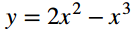我们可以在文件中生成这些分散的点：dataset_1D.dat：# x y 0 0 1 1 2 0 3 -9 4 -32 以下是这些点的一维插值代码：加载这些分散的点 创建x_mesh 执行1D插值 代码： ^{pr2}$

图中显示了以下内容：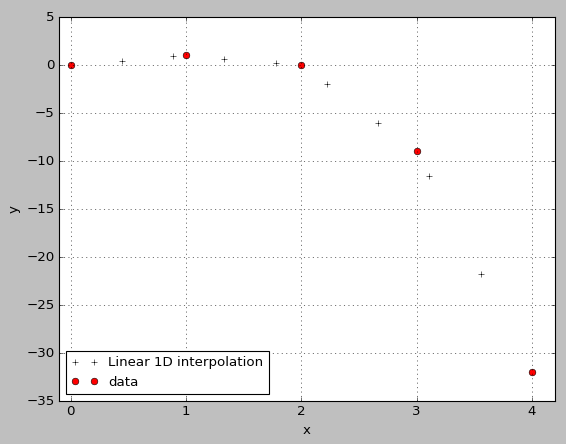现在，考虑这个z(x，y)函数：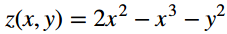我们可以在文件中生成这些分散的点：dataset_2D.dat：# x y z

0 0 0

1 1 0

2 2 -4

3 3 -18

4 4 -48

在这种情况下，我们必须执行二维插值：import numpy as np

from scipy.interpolate import interp1d, interp2d, interpnd

import matplotlib.pyplot as plt

from mpl_toolkits.mplot3d import Axes3D

# Load the data:

x, y, z = np.loadtxt('./dataset_2D.dat', skiprows = 1).T

# Create the function Z_inter for interpolation:

Z_inter = interp2d(x, y, z)

# Create the x_mesh and y_mesh :

x_mesh = np.linspace(1.0, 4, num=10)

y_mesh = np.linspace(1.0, 4, num=10)

print x_mesh

print y_mesh

# We calculate the z-interpolated of this x_mesh and y_mesh :

Z_interpolated = Z_inter(x_mesh, y_mesh)

print Z_interpolated

print type(Z_interpolated)

print Z_interpolated.shape

# plot:

fig = plt.figure()

ax = Axes3D(fig)

ax.scatter(x, y, z, c='r', marker='o')

plt.legend(['data'], loc='lower left', prop={'size':12})

ax.set_xlabel('x')

ax.set_ylabel('y')

ax.set_zlabel('z')

plt.show()

图中显示了以下内容：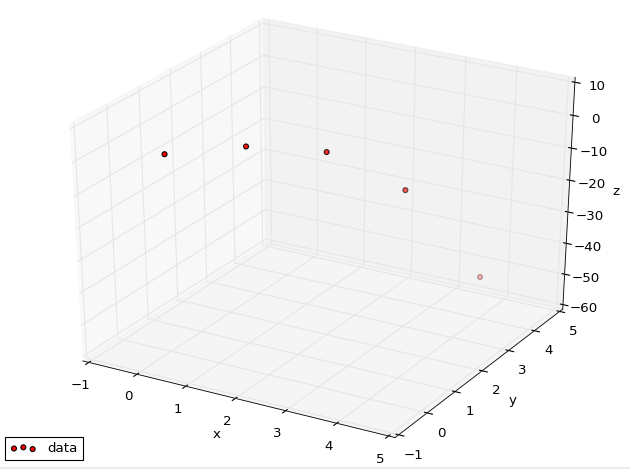其中，分散的数据再次以红点显示，以与二维图一致。在我不知道如何解释Z_interpolated结果：

根据上面代码的打印线，

Z_interpolated是一个n维numpy数组，形状为(10,10)。换句话说，是一个有10行10列的二维矩阵。

对于x_mesh[i]和{}的每个值，我都希望得到一个插值的z[i]值，为什么我没有收到这个？在我怎样才能在3D绘图中绘制插值数据(就像2D绘图中的黑色十字)？在

展开全文• 此函数用内插值或相邻值替换一维数组中的 NaN 值。 插值方案假设数据是均匀分布的。 此函数不进行外推。 句法x = repnan(x); x = repnan(x,method); 描述x = repnan(x) 返回 x 无 NaN。 x = repnan(x,method) ...matlab
• •XI 可能是个 n-dim 数组•Y 可以是列向量或矩阵（如在 INTERP1Q 中） • 如果Y 是矩阵，而XI 是N 维数组，则F 将有N+1 维。 前N维对应size(XI)，N+1维对应size(Y,2)。 •F=LERP(X,Y,XI,EXTRAP) 将扩展函数域...matlab
• 做完之后，大师说这个程序还有优化的空间，因为计算机的内存时一块一维连续的空间，如果这里出发采用一维数组去实现，效果会更好，插2次值就OK，而且每次插值采用的时间复杂度为O(n)。同时涉及到一维数组及二维数组...

## 1.1前言

1.本文是博主本着虚心学习的态度与大家交流，博文难免会有错误之处，希望大家指正；
2.本文是针对对C语言以及图像放大的基础讨论，如是大牛们可以直接忽略本文；
3.运行环境：由于在不同电脑配置和系统上的运行时间有差异，本程序的测试平台：电脑CPU为Intel奔腾双核E6500，主频2.93GHZ内存4GB.虚拟机为10.0版的VMware，linux系统为Fedora16

要讲程序的运行速度，还是要从计算机的底层实现为出发点，以下一个图像的两倍线性放大论证此事实。刚刚接触图像处理，要完成图像的两倍线性放大，一想到图像有长与宽，最先想到用二维数组来实现，每次插值采用时间复杂度为O(n^2)的两个for循环来实现，插几次值就OK了。做完之后，大师说这个程序还有优化的空间，因为计算机的内存时一块一维连续的空间，如果这里出发采用一维数组去实现，效果会更好，插2次值就OK，而且每次插值采用的时间复杂度为O(n)。同时涉及到一维数组及二维数组的在堆的动态内存分配，以及以一位数组、二维数组作为函数的形参，进行参数传递。

主旨: 采用隔行隔列插入，左右及上下取均值把640x480的图像放大2倍，变成1280x960的图像，测试程序运行效率时选择了稍大点的数据，效果会比较明显。

## 2.1插值函数参数为二维数组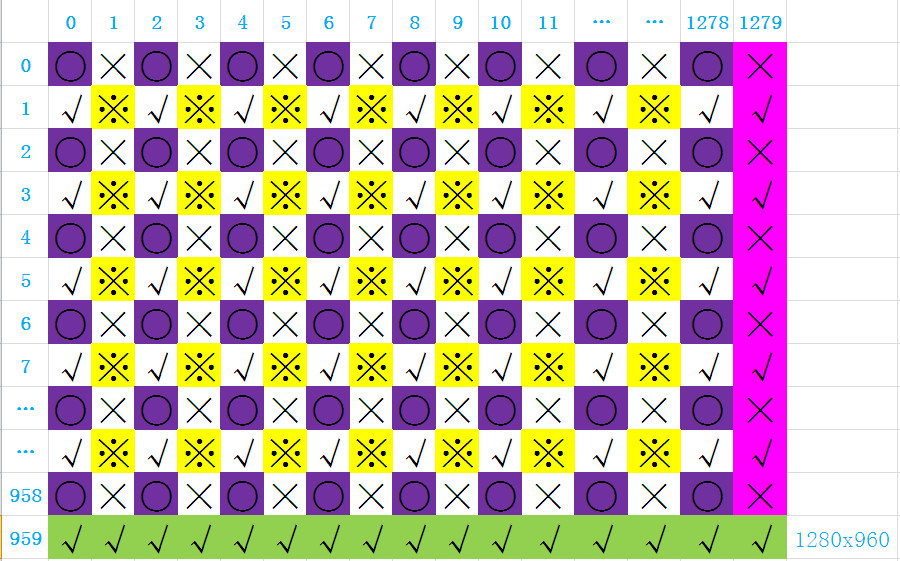紫色框的圆代表原始数据，首先上下取均值插(√)，再左右取均值插(×)，再取四个均值插(※)，最后插最后一列和最后一行。数据来源于计算机生成的伪随机数，为了方便测试该程序，改用较大分辨率2048x1536（现实没见到这种分辨率，按4:3的比例赋的值，测试用即可）程序从linux平台考过来的，格式可能会有一些乱。

源码如下：

#include <stdio.h>
#include <string.h>
#include <stdlib.h>
#include <ctype.h>
#include <sys/time.h>
typedef unsigned short dbyte;//图像每个像素点为双字节（双char型），且都大于零；char为8bit，short为16bit，用short来表示双char

#define H 1536  //表示图像分辨率的高度
#define W 2048  //表示图像分辨率的宽度

int interpolation_expansion(dbyte **original_data, dbyte **processed_data);//插值函数声明

int main(int argc, int *argv[])
{
int i, j;
dbyte **input_data, **output_data;
//内存分配
input_data = (dbyte **)malloc(H*sizeof(dbyte *));//为二维数组H行分配dbyte*内存
for(i = 0; i < H; i++)
{
input_data[i] = (dbyte *)malloc(W*sizeof(dbyte));//为每行中应放存放多少个dbyte元素分配内存
memset(input_data[i],0,W);//初始化内存
}
output_data = (dbyte **)malloc(2*H*sizeof(dbyte *));
for(i = 0; i < 2*H; i++)
{
output_data[i] = (dbyte *)malloc(2*W*sizeof(dbyte));
memset(output_data[i],0,2*W);
}
for(i = 0;i < H; i++)
{
for(j = 0; j < W; j++)
{
input_data[i][j] = rand();//数据采用伪随机数
}
}
for(i = 238; i < 241; i++)
{
for(j = 320; j < 324; j++)
printf("[%d][%d]_ %d  ",i , j, input_data[i][j]);
printf("\n");
}
struct timeval tpstart, tpend;
float timeuse;
gettimeofday(&tpstart, NULL);//获取函数调用之前的时间
interpolation_expansion(input_data, output_data);
gettimeofday(&tpend, NULL);//获取函数调用结束的时间
printf("_________________________<_________>_________________________\n");
for(i = 476; i < 481; i++)
{
for(j = 640; j < 648; j++)
printf("[%d][%d]_%d  ", i, j, output_data[i][j]);
printf("\n");
}
//计算函数调用多花费的时间，单位为秒
timeuse = 1000000*(tpend.tv_sec - tpstart.tv_sec)+      tpend.tv_usec - tpstart.tv_usec;
timeuse /= 1000000;
printf("The usetime TWO-dimensional array is %fS\n",timeuse);

//释放内存，【好像不用循环直接free(input_data)也可以，前提是需要知道第二维，我不是很确定】
for(i = 0; i < H; i++)
{
free(input_data[i]);
}
for(i = 0; i < 2*H; i++)
{
free(output_data[i]);
}
}

int interpolation_expansion(dbyte **original_data, dbyte **processed_data)//插值函数的原型
{
int i, j;
int last_line = 2*H -1;
int last_column = 2*W -1;
short tmp_data;

//set values for expansion array 把原来图片的像素点数据插入到需要放大的图片上（下标[偶数][偶数]标示○）
for(i = 0;i < H; i++)
{
for(j = 0; j < W; j++)
{
processed_data[2*i][2*j] = original_data[i][j];
}
}

//insert values into that subscript is odd line caculating by up number and down number用已知值进行上下取均值插入(下标[偶数][奇数]标示√)
for(i = 1; i < 2*(H-1); i += 2)//height_line
{
for(j = 0;j < 2*W; j += 2)//width_column
{

processed_data[i][j] =  (processed_data[i-1][j] +  processed_data[i+1][j])/2;
}
}

//insert values into that subscript is odd column caculating by left number and right number左右取均值插入(下标[奇][偶]标示×)
for(i = 0; i < 2*H; i += 2)//height_line
{
for(j = 1;j < 2*(W-1); j += 2)//width_column
{

processed_data[i][j] =  (processed_data[i][j-1] +  processed_data[i][j+1])/2;
}
}

//insert values into that both weight and height are odd numbers 对周围四个取均值（下标[奇][奇]标示※）
for(i = 1; i < 2*(H-1); i += 2)//height_line
{
for(j = 1; j < 2*(W-1); j += 2)
{
processed_data[i][j] = (processed_data[i-1][j-1] + processed_data[i-1][j+1] + processed_data[i+1][j-1] + processed_data[i+1][j+1])/4;

}
}
//insert values into the last line and column对最后一行和最后一列进行插值
for(i = 0; i< 2*H-1; i++)//insert values into last colum
{
if((tmp_data = 2*processed_data[i][last_column-1]-processed_data[i][last_column-2])>0)
{
processed_data[i][last_column] = tmp_data;
}
else
{
processed_data[i][last_column] = processed_data[i][last_column-1];
}
}
for(j = 0; j< 2*W-1; j++)//insert values into last line
{

if((tmp_data = 2*processed_data[last_line-1][j] - processed_data[last_line-2][j]) > 0)
{
processed_data[last_line][j] = tmp_data;
}else
{
processed_data[last_line][j] = processed_data[last_line-1][j];
}
}
processed_data[last_line][last_column] = (processed_data[last_line-1][last_column] + processed_data[last_line][last_column-1] + processed_data[last_line-1][last_column-1])/3;
return 1;
}

本段程序实现了函数形参为二维数组的传递，以及二维数组在堆的动态内存分配。我当初直接为二维数组在栈上分配空间，也就是指定死二维数组的行和列，但是当分辨率较高时，在linux编译能通过，但是运行遇到段错误（吐核），如下目前也没有找到其根本原因，后面用二维数组的动态内存分配解决了这个问题……

## 2.2程序的测试结果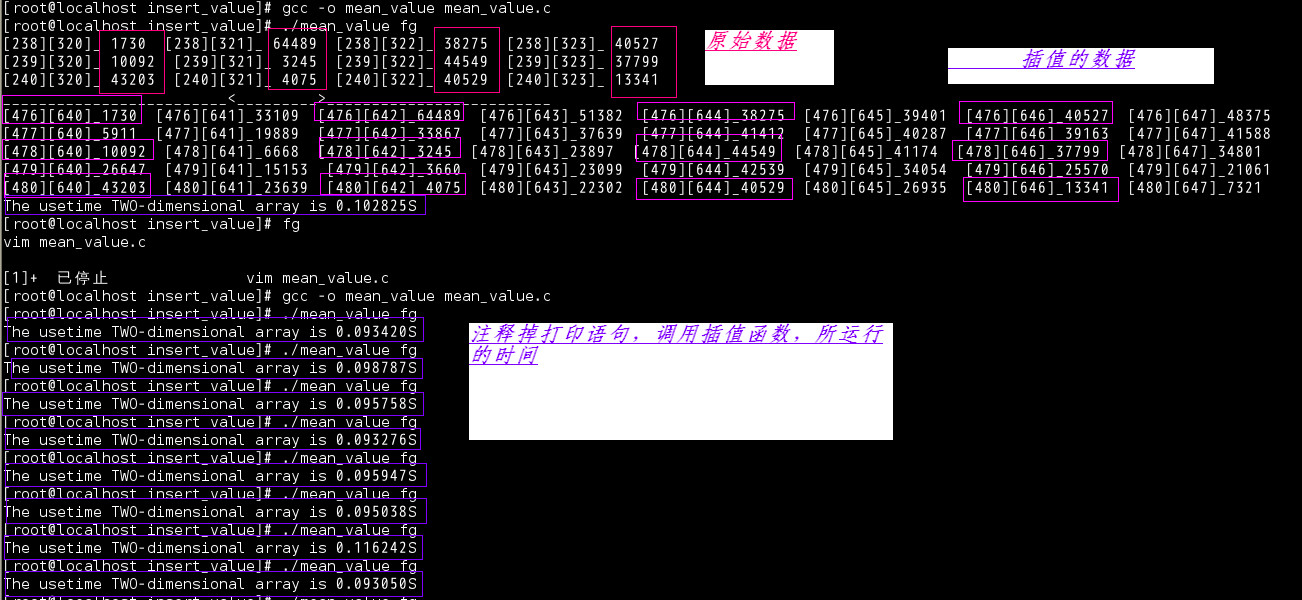截取的数据为放大前、后图片中间的某块数据，平均运行时间为0.098260秒

## 3.1插值函数参数为一维数组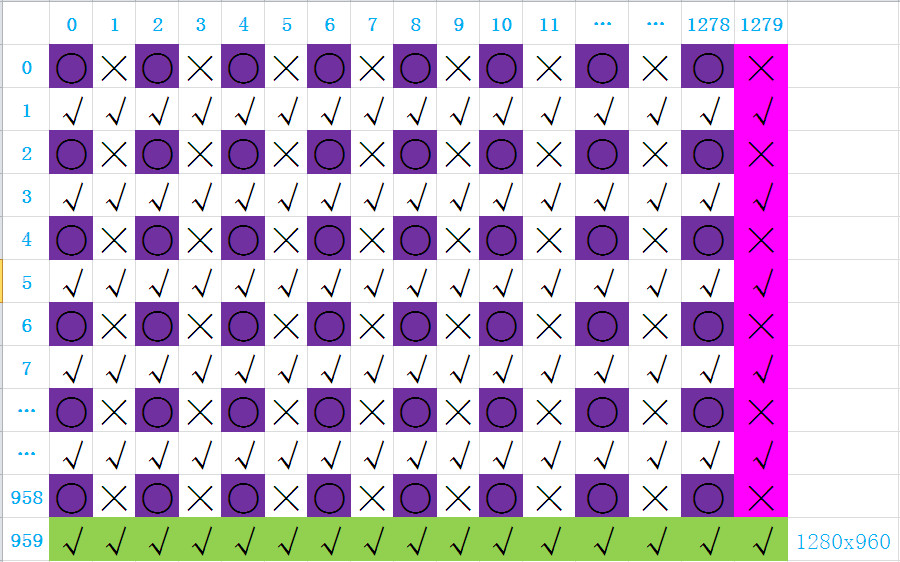紫色框的圆代表原始数据，2个for循环实现，首先左右取均值插值(×)，再上下取均值插值(√)；也可以在插值子函数里实现动态内存，但是为了方便管理，尽量在主函数分配，谁用谁分配和释放，只需要调用函数接口即可。

源码如下：

#include <stdio.h>
#include <string.h>
#include <stdlib.h>
#include <ctype.h>
#include <sys/time.h>

typedef unsigned short dbyte;
#define M 1536  //原始图片高度
#define N 2048  //原始图片宽度
#define H 3072    //height  放大2倍图片的高度
#define W 4096   //weight  放大2倍图片的宽度

dbyte* insert_values(dbyte *original_data, dbyte *processed_data);

int main(int argc, int *argv[])
{
int i, count;
//内存分配
dbyte *original_data = (dbyte *)malloc(M*N*sizeof(dbyte));
dbyte *processed_data = (dbyte *)malloc(W*H*sizeof(dbyte));
if(original_data == NULL)
{
printf("please allocate the input memery again!\n");
}

if(processed_data == NULL)
{
printf("please allocate the output memery again!\n");
}
//初始化内存为零
memset(original_data,0,M*N);
memset(processed_data,0,W*H);

//the test program as the follows.
//set original data
for(i  = 0; i < N*M; i++)
{
original_data[i] = rand();
}

//printf have not be to insert
for(i = 238*N + 320, count = 1; i < 324 + 241* N; )
{
printf(" %d__%ld   ",i, original_data[i]);
if(count%4 == 0)
{
count = 1;
i += (N-3);
printf("\n");
}else{
count++;
i++;
}
}
printf("______________*****************_____________________________*****************__________\n");

struct timeval tpstart, tpend;
float timeuse;
gettimeofday(&tpstart, NULL);
insert_values(original_data,processed_data);//use of the function
gettimeofday(&tpend, NULL);

//printf insert have been down
for(i = 476*W + 640, count = 1; i < 648 + 481* W; )
{
printf(" %d__%ld   ", i, processed_data[i]);
if(count%8 == 0)
{
count = 1;
i += (W-7);
printf("\n");
}else{
count++;
i++;
}
}
timeuse = 1000000*(tpend.tv_sec - tpstart.tv_sec)+ tpend.tv_usec - tpstart.tv_usec;
timeuse /= 1000000;
printf("The  usetime of One-dimensional array is %fS\n",timeuse);
free(processed_data);
free(original_data);
}

dbyte* insert_values(dbyte *original_data, dbyte *processed_data)//The value of return can be define by yourself
{
int i, j;
short tmp;
//set_values from original_data to the processed_data
for(i = j = 0; i<W*(H-1); i += 2, j++)//i为循环要放大的图像，步长为2（隔列插入），j用来循环遍历原始数据
{
if((i!= 0) && (i%W) == 0)//判断是否把一个W宽度的数据插完，真就跳W个数据继续插值，注意：如果不添加i!=0，当i=0，只判断i%W==0，最开始的W个数据时没有的
{
i += W;
}
processed_data[i] = original_data[j];
}

//insert_values into the line caculating by left number and right number 左右均值插值
for(i = 1;i < W*(H-1); i += 2)
{
if((i+1)%W == 0)//最后一列的插值方法，图像由左向右延伸
{
if((tmp = processed_data[i-1]*2-processed_data[i-2]) > 0) //如果中间值的2倍大于两边值之和，就插2倍中间值减去已知的值
{
processed_data[i] = tmp;
}
else{
processed_data[i] = processed_data[i-1];//如果需要插的值是小于零的，直接复制前一个像素点数据
}
i += W;
}
else
{
processed_data[i] = (processed_data[i-1] + processed_data[i+1])/2;

}
}

//insert_values into the line caculating by up number and down number
for(i = W; i < W*H; i++)
{
if(i < W*(H-1))
{
processed_data[i] = (processed_data[i-W] + processed_data[i+W])/2;
if((i+1)%W == 0)
{
i += W;
}
}else//最后一行的插值方法，图像由上向下延伸
{
if((tmp = processed_data[i-W]*2-processed_data[i-2*W]) > 0)
{
processed_data[i] = tmp;
}else{
processed_data[i] = processed_data[i-W];
}
}
}
return processed_data;
}

## 3.2一维数组程序的测试结果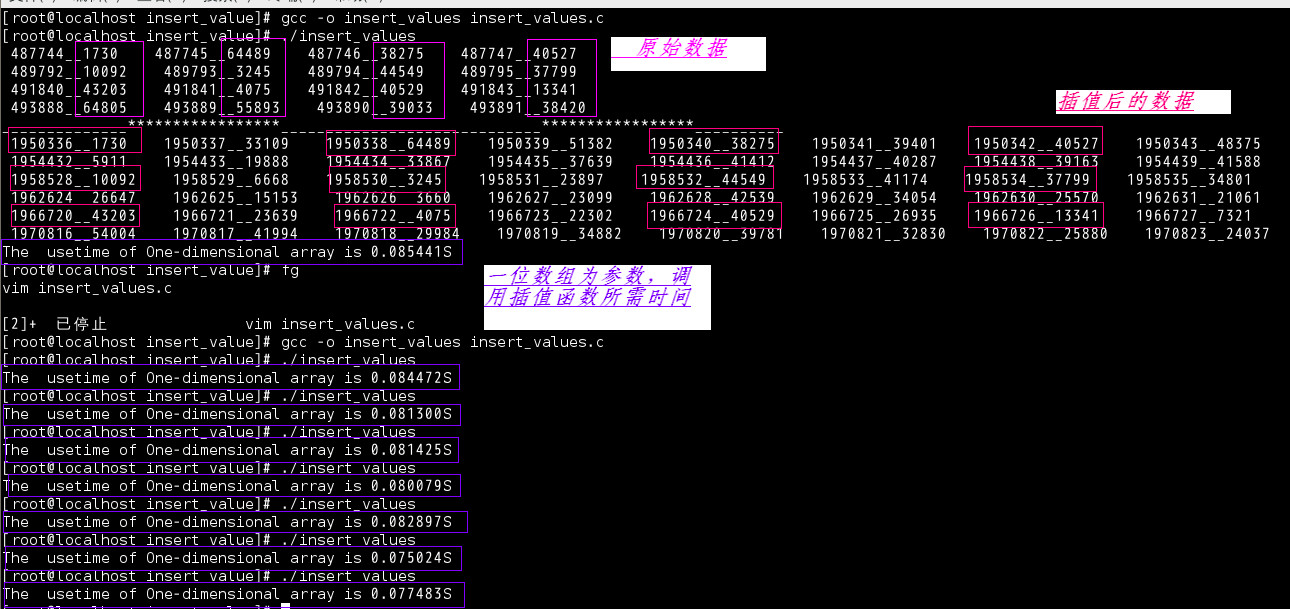截取的数据为放大前、后图片中间的某块数据，平均运行时间为0.081015秒

## 4.1结论

(0.098260-0.081015)=0.17245秒
0.17245/0.09826*100=17.55%,效率提高了百分之17.55。可能有的人觉得没有必要，毕竟现在的CPU处理速度已经相当快了。但如果是在嵌入式设备上就不一定了，毕竟嵌入式设备的资源都是有限的，所以很宝贵，充分利用它才是王道。

展开全文一维数组 二维数组 动态内存分配
• ## 一维插值

千次阅读 2020-06-19 19:04:50
插值 拉格朗日方法 代码如下： function y0=Lagrange(x,y,x0) n=length(x); l=ones(1,n); y0=0; for j=1:n for k=1:n if j~=k l(j)=l(j)*(x0-x(k))/(x(j)-x(k)); end end end for i=1:n y0=y0+l(i)*y(i); end ...matlab
• 三个参数都是数组或列表,并且个数相等,你可以把它们想想成二图像中的一些点,第个参数可以是个数,也可以是组数,就是从后面两个数组中进行插值得到线性插值 这个案例中下x2就是个插值点. x3是进行了...线性插值
• (我想完全用this但是用Java做)这是一张图片：基本上我有每个点的x和y坐标以及与每个点相关的z值,我想在它们之间进行插值并填充图像的中心.使用Java执行此操作的最佳方法是什么？我可以使用内置的2D插值库吗？或者我...
• python-在numpy数组中插值NaN值有没有种快速的方法用(例如)线性插值替换numpy数组中的所有NaN值？例如，[1 1 1 nan nan 2 2 nan 0]将被转换成[1 1 1 1.3 1.6 2 2 1 0]9个解决方案89 votes让我们首先定义个简单的...
• 1. 简介NumPy(Numerical Python) 是 Python 语言的个扩展程序库，支持大量的维度数组与矩阵运算，此外也针对数组运算提供大量的数学函数库。最主要的数据结构是ndarray数组。NumPy 通常与 SciPy(Scientific Python...
• ## 二维线性插值方法

千次阅读 2021-12-21 22:57:08
​ 前几天在进行数据仿真的时候,对于将表格离散数据转化成连续数据一直是件十分棘手的事情，在网站上找了许多资源最后才找到可以利用二维线性插值的方法将数据进行转化。 1.原理 ​ 是要将m×nm\times nm×n的二...机器学习 人工智能
• ## C# 线性插值

千次阅读 2021-01-01 11:51:05
1. 对于给定起点、终点和插值点数目，生成线性插值后的数组 2.对于缺失部分值得数据序列，进行线性插值，补充该序列（如有组数据中间缺失了部分，用其起点和终点进行线性插值
• 尽管 numpy 自身提供了 numpy.interp 插值函数，但只能做一维线性插值，因此，在实际工作中，我们更多地使用 scipy 的 interpolate 子模块。关于 numpy 和 scipy 的关系，有兴趣的话，可以参考拙作《数学建模三剑客...三维插值
• C++实现复数的一维线性插值interp1导言函数需求分析源码注意 导言 最近在进行Qt开发，涉及大量的matlab转C的工作，其中包括插值滤波等，这里对matlab的interp1函数进行了研究并用C++进行了重写。 经对比，结果与...matlab
• x0=[0 3 5 7 9 11 12 13 14 15 ]; y0=[0 1.2 1.7 2.0 2.1 2.0 1.8 1.2 1.0 1.6 ]; x=0:0.1:15; y1=interp1(x0,y0,x); y2=interp1(x0,y0,x,'spline'); subplot(2,1,1) plot(x0,y0,'k+',x,y1,'r') ...matlab
• ## Python_线性插值

千次阅读 2022-04-21 09:53:25python
• 文章目录、认识插值二、拉格朗日插值三、分段线性插值四、三次样条插值 、认识插值 插值的分类： 拉格朗日插值（高次多项式插值）： 其插值函数在整个区间上是个解析表达式，便于再次开发利用； 曲线光滑...
• import numpy as np ...from scipy.interpolate import interp1d #引入scipy中的一维插值库 from scipy.interpolate import griddata#引入scipy中的二维插值库 x = np.linspace(0, 10, num=11, endpoint...
• 在算法分析过程中，我们经常会遇到数据需要处理插值的过程，为了方便理解，我们这里给出相关概念和源程序，希望能帮助到您!案例：已知坐标 (x0, y0) 与 (x1, y1)，要求得区间 [x0, x1] 内某一点位置 x 在直线上的y...
• MATLAB 提供了 interp1(x,y,xq,'Method')函数命令可以进行一维插值，其中一维插值有四种常用的方法，也就是 ‘Method’ 可以选择邻近点插值Nearest，线性插值Linear，三次样条插值Spline和立方插值Pchip。...matlab
• 线性插值 先介绍线性插值的概念。 已知两个点(x1, y1)、(x2, y2)，求它们中间横坐标为x的点的y值。 则可以利用如下公式进行插值计算。其中a和(1-a)为x距离x1和x2的距离占(x2-x1)的比例。 y = a*y1 + (1-a)*y2...数字图像处理
• 函数功能：输入两个值，制定插值后数组长度，输出线性插值后的数组。 void test(double a, double b){ cout << "section: " << a << " " << b << endl; const int ...
• 利用Matlab实现对矩阵行或列的任意次插值（重采样）matlab
• 拉格朗日插值法： 可以用于少样本点的情况，一定可以返回较好的插值函数。当样本点较多时，函数复杂度急剧上升。 样条插值法： 低阶多项式进行拟合，误差比拉格朗日稍大，但是避免了龙格现象。 ...matlab python...